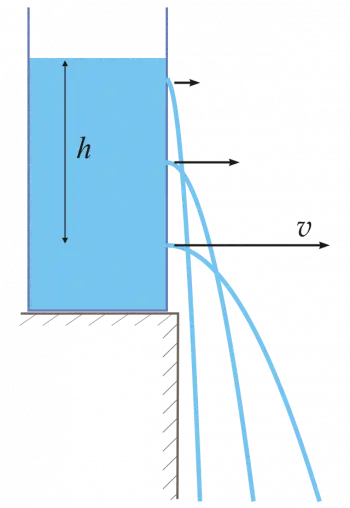# Torricelli's Law: Experiment and Examples of the TheoremTorricelli's theorem is a law of fluid mechanics that studies fluid behavior in a container with a hole.

Torricelli's law is used in hydraulics and fluid mechanics to determine the flow rate of a liquid through an orifice. Torricelli's law can generally be applied in different areas, such as civil engineering, hydrology, physics, and meteorology.

This law was enunciated by the Italian physicist and mathematician Evangelista Torricelli in the 17th century.

## Definition of Torricelli's Law

Torricelli's law states that the speed of a liquid flowing through the hole of a container is equal to the velocity acquired by a body that falls freely from a height equal to the distance from the level of the liquid to the center of gravity of the container's orifice.

The characteristics of the fluid for the theorem to be valid is that the fluid must be incompressible, with zero viscosity, and must be subjected only to the gravitational force.

This theorem is based on the law of conservation of mechanical energy, which states that the total energy of a closed system is conserved. Specifically, it is a consequence of Bernoulli's principle.

## Examples of Torricelli's Law

Torricelli's law can be observed in a wide variety of practical situations. Here are some examples:

1. A Pitot tube: This instrument measures the speed of air or the flow of liquids in a conduit. The Pitot tube consists of two tubes, one of which measures the static pressure of the fluid and the other measures the total pressure, which includes both static and dynamic pressure (caused by the velocity of the liquid). The pressure difference between the two tubes can be used to calculate the speed of the fluid using Torricelli's theorem.

2. Ornamental fountains: Some decorative fountains work by spouting water through a nozzle at the top of a container. The water is pushed up by the pressure of the water in the container and then falls back into the container. The velocity of the water leaving the nozzle can be calculated using Torricelli's law, using the height of the water column above the nozzle.

3. Bottle Filling: When filling a bottle or container with liquid from a larger container, the liquid flow slows as it approaches the liquid level in the bottle. This is because the pressure of the fluid in the large container is transmitted to the liquid in the bottle, and the velocity of the liquid leaving the bottle can be calculated using Torricelli's law, using the height of the liquid in the large container.

## Experiment to Show Torricelli's Law

Here we show a straightforward experiment to illustrate how Torricelli's theorem works. This experiment can be done with materials that can be found at home.

### Materials:

• An empty plastic bottle

• A sharp nail or needle

• Water

### Instructions:

1. Fill the plastic bottle halfway with water.

2. Punch a small hole in the middle of the bottle with a nail or needle.

3. Place a container under the bottle to catch the water from the spot.

4. Open the bottle cap to allow air in and water to flow out smoothly.

### Observations:

When the hole is made in the bottle, water starts to flow through the hole. The speed of the water decreases as more water leaves the bottle. The water particles coming out of the hole describe the typical trajectory of a parabolic shot with a horizontal initial velocity.

Knowing the height of the hole concerning the surface of the container where the water falls and the horizontal distance from the hole to the point where the water falls, we can calculate the exit velocity of the water at any instant.

Author:

Published: April 24, 2023
Last review: April 24, 2023